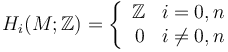# Homology sphere

## Definition

Suppose$n$ is a natural number. A homology$n$-sphere is a$n$-dimensional manifold whose homology groups (over the ring of integers$\mathbb{Z}$) match those of the sphere. Specifically, a manifold$M$ is a homology sphere if its homology groups are as follows:$H_i(M; \mathbb{Z}) = \left \lbrace \begin{array}{rl} \mathbb{Z} & i = 0,n \\ 0 & i \ne 0, n \end{array}\right.$

Note that it is important to explicitly specify that the manifold is$n$-dimensional, otherwise a cylinder over a sphere would satisfy the definition.

Note that we exclude the case$n = 0$ from consideration.

## Examples

For any positive integer$n$, the$n$-sphere$S^n$ is a homology sphere.

For$n = 3$, the Poincare homology sphere, obtained as the quotient of the 3-sphere by the binary icosahedral group (that is isomorphic to$SL(2,5)$) is a homology sphere.

## Facts

### Complement of a point

The complement of any point in a homology sphere is an acyclic space. In particular, when the homology sphere is not a sphere (and specifically, its fundamental group is a nontrivial perfect group) then the complement of a point in it is acyclic but not weakly contractible.

### Suspension

• The suspension of any homology 3-sphere that is not homeomorphic to the 3-sphere is a homology manifold that is not a manifold.

## Relation with other properties

### Weaker properties

Property Meaning Proof of implication Proof of strictness (reverse implication failure) Intermediate notions
rational homology sphere manifold whose homology groups over rationals match those of a sphere of the same dimension |FULL LIST, MORE INFO
For$n > 1$, space with perfect fundamental group
For odd$n$, space with Euler characteristic zero